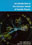© CAMBRIDGE UNIVERSITY PRESS 1998

1.10 Units

In particle physics it is usual to simplify the appearance of equations by using units in which= 1 and c = 1. In electromagnetism we set0 = 1 (so that the force between charges q1 and q2 is q1q2 / 4r2), and µ0 = 1, to give c2 = (µ00)-1 = 1. We shall occasionally reinsert factors ofand c where it may be reassuring or illuminating, or for the purposes of calculation. It is useful to remember thatc197 MeV fm, e2 / 41.44 MeV fm,= e2 / 4c(1/137), c3 x 1023 fm s-1.

Energies, masses, and momenta are usually quoted in MeV or GeV, and we shall follow this convention.